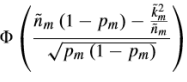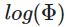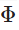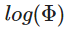# Cannot perform the operation: {real affine} .* {convex}

Hi.I writting my optimization problem in cvx and running that but i had a error .And I think the error is in the following part of the optimization problem:where m,p are constants and m=3. k(3,1) and n(3,1) are the variables.
How can I express it in a DCP-compliant manner?
normcdf(((sqrt(n(m))(1-p(m))-k(m)inv_pos(sqrt(n(m)))))/sqrt(p(m)(1-p(m))), 0, 1)
What’s wrong in the expression given above?I get the error that"Disciplined convex programming error:
Cannot perform the operation: {real affine} .
{convex}"
please if it is possible help me…

You are not following the DCP rules.

I think you can handle an approximation of the log of \Phi as a concave function of `k` and `n` (I don’t understand exactly how you are dealing with the vector aspect of `k` and `n`, so I 'll leave those details to you).

Use `quad_over_lin` for `k^2/n`, which will result in a concave argument of \Phi. Then use log_norm_cdf for the log of \Phi. If you need \Phi instead of log(\Phi), you are out of luck.

help log_normcdf

log_normcdf Logarithm of the cumulative normal distribution.
Y = log_normcdf(X) is the logarithm of the CDF of the normal
distribution at the point X.

``````                             1    / x
log_normcdf(X) = LOG( ------- |   exp(-t^2/2) dt )
sqrt(2) / -Inf

For numeric X, log_normcdf(X) is computed using the equivalent
expression LOG(0.5*ERFC(-X*SQRT(0.5))). When X is a CVX variable, a
approximation gives good results when -4 <= x <= 4, and will be
improved in future releases of CVX.

For array values of X, the log_normcdf returns an array of identical
size with the calculation applied independently to each element.

X must be real.

Disciplined convex programming information:
log_normcdf is concave and nondecreasing in X. Therefore, when used
in CVX specifications, X must be concave.``````

Oh thank you so much.The quad_over_lin and log_normcdf is very helpful to me,and this optimization problem can be solved by cvx if there is.But sir,if I usehere,is this problem not solved by cvx?

If you can’t use log(\Phi), then you can not handle it in CVX.

If \Phi is an objective function to be maximized, then you can maximize log(\Phi) instead. If you have a constraint \Phi \ge constant, you can change that to log(\Phi) \ge log(constant).

OK I got it.I will replacewithin this optimization problem.Thank you so much,sir.